# KSEEB Solutions for Class 7 Maths Chapter 14 Symmetry Ex 14.1

Students can Download Chapter 14 Symmetry Ex 14.1, Question and Answers, Notes Pdf, KSEEB Solutions for Class 7 Maths, Karnataka State Board Solutions help you to revise complete Syllabus and score more marks in your examinations.

## Karnataka State Syllabus Class 7 Maths Chapter 14 Symmetry Ex 14.1

Question 1.
Copy the figures with punched holes and find the axes of symmetry for the following :Solution: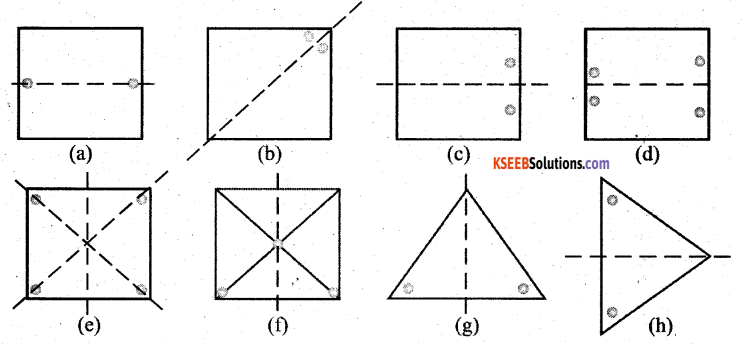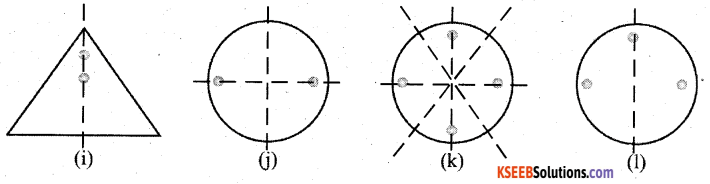The dotted lines are the axes of symmetry for the above figs.

Question 2.
Given the line(s) of symmetry, find the other hole(s) :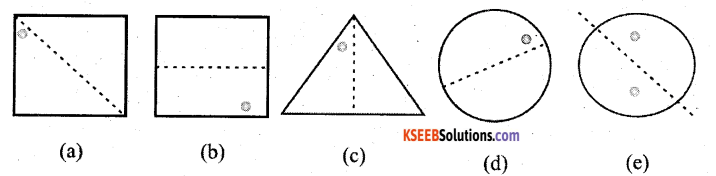Solution: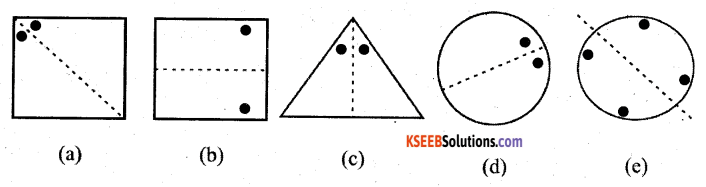Question 3.
In the following figures, the mirror line (i.e., the line of symmetry) is given as a dotted line. Complete each figure performing reflection in the dotted (mirror) line. (You might perhaps place a mirror along the dotted line and look into the mirror for the image). Are you able to recall the name of the figure you complete ?
Solution: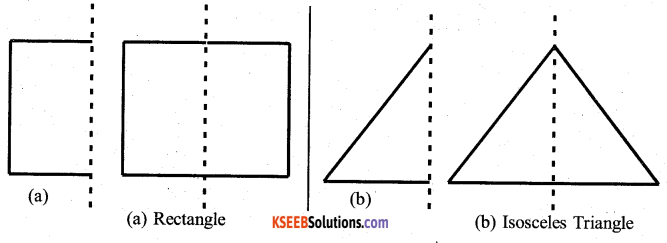Solution: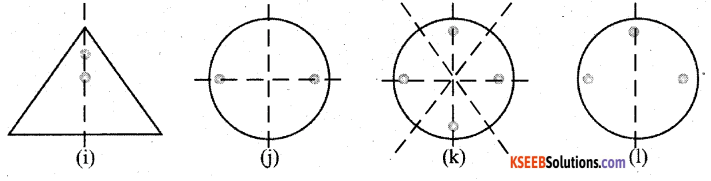The dotted lines are the axes of symmetry for the above figs.

Question 2.
Given the line(s) of symmetry, find the other hole(s) :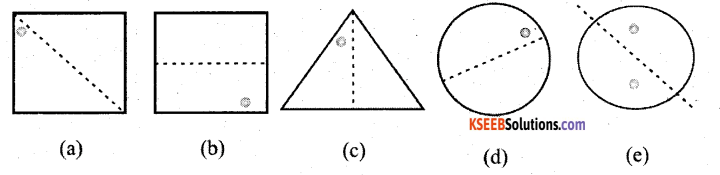Solution:Question 3.
In the following figures, the mirror line (i.e., the line of symmetry) is given as a dotted line. Complete each figure performing reflection in the dotted (mirror) line. (You might perhaps place a mirror along the dotted line and look into the mirror for the image). Are you able to recall the name of the figure you complete ?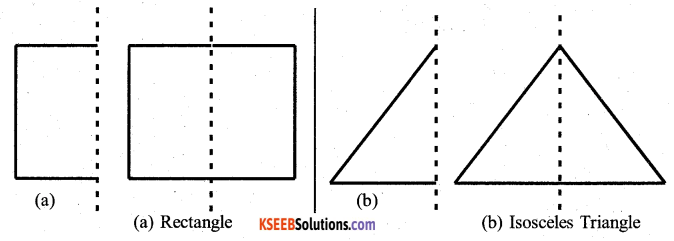Solution: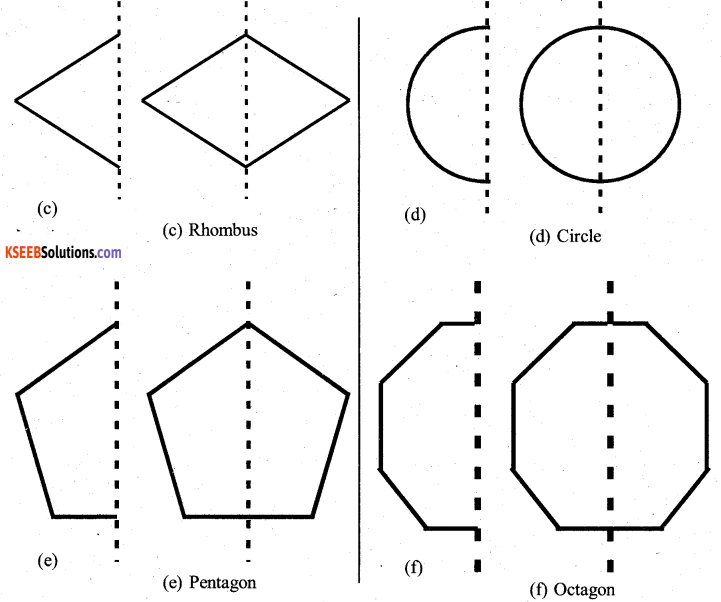Question 4.
The following figures have more than one line of symmetry. Such figures are said to have multiple lines of symmetry.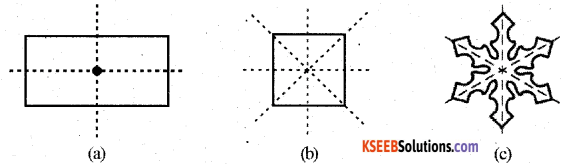Identify multiple lines of symmetry, if any, in each of the following figures:
a)Solution: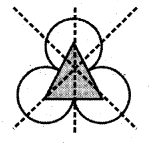b)Solution:c)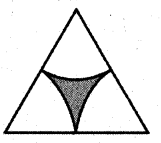Solution: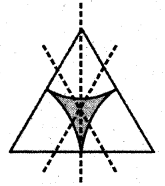d)Solution: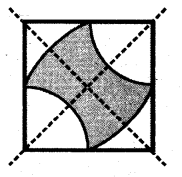e)Solution:f)Solution: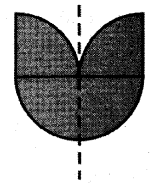g)Solution:h)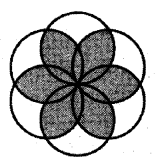Solution: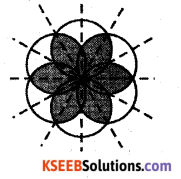Question 5.
Copy the figure given here :
Solution:
Take any one diagonal as a line of symmetry and shade a few more squares to make the figure symmetric about a diagonal. Is there more than one way to do that? will the figure be symmetric about both the diagonals?
Yes, there is more than one way to make the figure symmetric.i) Let us take the diagonal BD and shade the squares as shown in the figure to make the figure symmetric about BD.
ii) Similarly the figure is symmetric about the diagonals.
iii) The figure is symmetric about EF and GH also.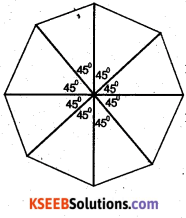Question 6.
Copy the diagram and complete each shape to be symmetric about the mirror line(s)
Take any one diagonal as a line of symmetry and shade a few more squares to make the figure symmetric about both the diagonals?
Solution: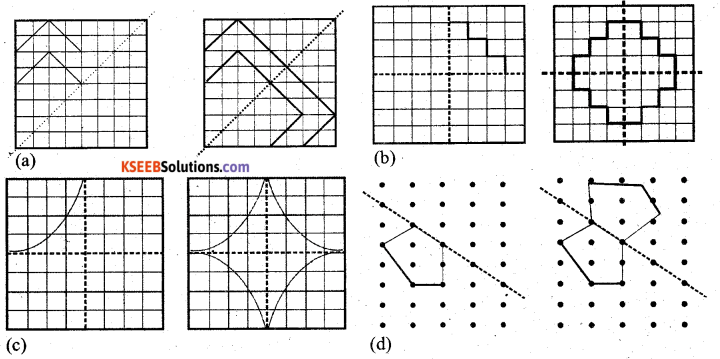Question 7.
State the number of lines of symmetry for the following figures.
Solution:
a)3 lines of symmetry.

b)1 line of symmetry

c)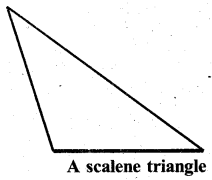Zero or No lines of symmetry.

d)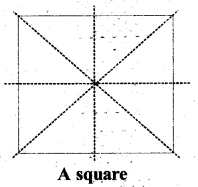4 lines of symmetry

e)2 lines of symmetry

f)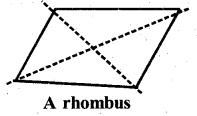2 lines of symmetry

g)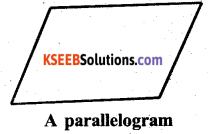Zero or No lines of symmetry

h)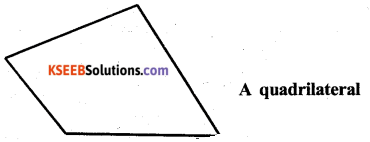Zero or No lines of symmetry

i)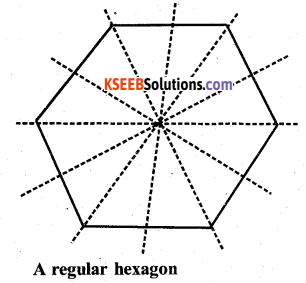6 lines of symmetry

j)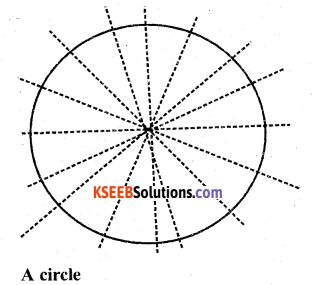Many or Infinite number of symmetry.

Question 8.
What letters of the English alphabet have reflectional symmetry (i.e. symmetry related to mirror reflection) about.
a. a vertical mirror
A H I M O T U V W X Y
These letter have vertical symmetry

b. a horizontal mirror
B C D E H I O X
These letter have horizontal symmetry.

c. both horizontal and vertical mirrors
H I O X
These have both horizontal and vertical symmetry

Question 9.
Give three examples of shapes with no line of symmetry.
Solution:
2) Scalene triangle
3) Parallelogram
Have no line of symmetry.Question 10.
What other names can you give to the line of symmetry of
a) an isosceles triangle?
b) a circle?
Solution:
a) The line of symmetry in an isosceles triangle is also known as Median.
b) The line of symmetry in a circle is also called Diameter.

error: Content is protected !!